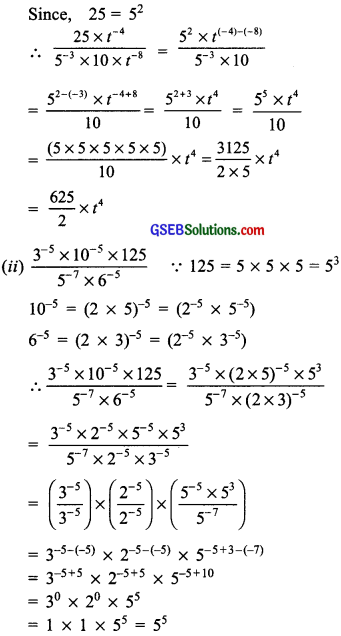# GSEB Solutions Class 8 Maths Chapter 12 Exponents and Powers Ex 12.1

Gujarat Board GSEB Textbook Solutions Class 8 Maths Chapter 12 Exponents and Powers Ex 12.1 Textbook Questions and Answers.

## Gujarat Board Textbook Solutions Class 8 Maths Chapter 12 Exponents and Powers Ex 12.1

Question 1.
Evaluate:
(i) 3-2
(ii) (-4)-2
(iii) ($$\frac{1}{2}$$)-5
Solution: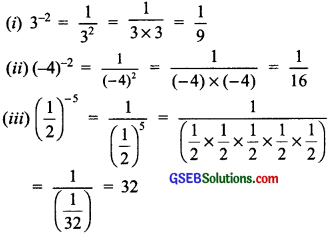Question 2.
Simplify and express the result in power notation with positive exponent?
(i) (-4)5 + (-4)8
(ii) $$\left(\frac{1}{2^{3}}\right)^{2}$$
(iii) (-3)4 × ($$\frac{5}{3}$$)4
(iv) (3-7 + 3-10) × 3-5
(v) 2-3 × (-7)-3
Solution:
(i) (-4)5 + (-4)8
∵ am + an = am-n
∴ (-4)5 + (-4)8 = (-4)5-8
= (-4)-3 = $$\frac{1}{(-4)^{3}}$$

(ii)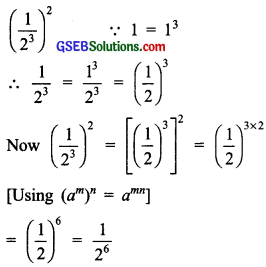(iii)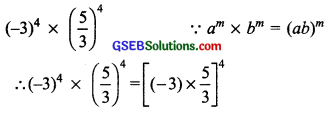= [(-1) × 5]4 = [(-1)4 × (+5)4]
= 1 × (5)4 = (5)4

(iv) (3-7 ÷ 3-10) × 3-5
∵ am + an = am-n and am × an = am+n
∴ (3-7 ÷ 3-10 × 3-5 = [3-7-(-10)] × 3-5
= 33+(-5) = 3-2 = $$\frac{1}{(3)^{2}}$$

(v) 2-3 × (-7)-3
∵ am × bm = (ab)m
∴ 2-3 × (-7)-3 = [2 × (-7)]-3
= [-14]-3 = $$\frac{1}{(-14)^{3}}$$Question 3.
Find the value of:
(i) (30 + 4-1) × 22
(ii) (2-1 × 4-1) + 2-2
(iii) ($$\frac{1}{2}$$)-2 + ($$\frac{1}{3}$$)-2 + ($$\frac{1}{4}$$)-2
(iv) (3-1 + 4-1 + 5-1)0
(v) {($$\frac{-2}{3}$$)-2}2
Solution:
(i) (30 + 4-1) × 22
∵ a0 = 1 and a-1 = $$\frac{1}{a}$$
∴ (30 + 4-1) × 22
= (1 + $$\frac{1}{2}$$) 22 = $$\frac{5}{4}$$ × 4 = 5

(ii) (2-1 × 4-1) ÷ 2-2
∵ am × bm = (ab)m
∴ (2-1 × 4-1) + 2-2 = (2 × 4-1) ÷ 2-2
= (21 × 22)-1 ÷ 2-2
= (21+2)-1) ÷ 2-2 = (23)-1 ÷ 2-2
= 2-3 ÷ 2-2 = (2)(-3)-(-2)
= (-2)-3+2 = 2-1 = $$\frac{1}{2}$$

(iii)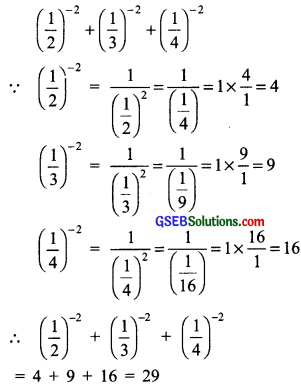(iv)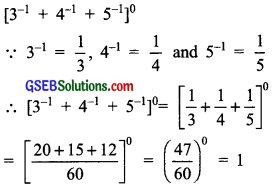(v)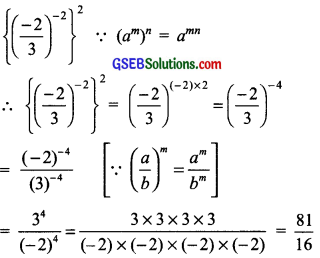Question 4.
Evaluate

1. $$\frac{8^{-1} \times 5^{3}}{2^{-4}}$$ and
2. (5-1 × 2-1) × 6-1

Solution:
1. $$\frac{8^{-1} \times 5^{3}}{2^{-4}}$$ = $$\frac{8^{-1} \times(5 \times 5 \times 5)}{2^{-4}}$$
= $$\frac{1}{8}$$ × 24 × 125 = $$\frac{1}{8}$$ × 2 × 2 × 2 × 2 × 125
= 2 × 125 = 250

2. (5-1 × 2-1) × 6-1) = (5 × 2-1) × 6-1)
= 10-1 × 6-1
= (10 × 6-1) = 60-1 = $$\frac{1}{60}$$Question 5.
Find the value of m for which 5m + 5-3 = 55.
Solution:
∵ am × bm = (ab)m
∴ L.H.S = 5m + 5-3
= 5m-(-3)
= 5m+3
Now, 5m+3 = 55
Since, bases 5 are equal, therefore exponents are also equal.
i.e; m + 3 = 5
or m = 5 – 3 = 2
Thus, the required value of m is 2.

Question 6.
Evaluate:
(i) $$\left\{\left(\frac{1}{3}\right)^{-1}-\left(\frac{1}{4}\right)^{-1}\right\}^{-1}$$
(ii) $$\left(\frac{5}{8}\right)^{-7} \times\left(\frac{8}{5}\right)^{-4}$$
Solution: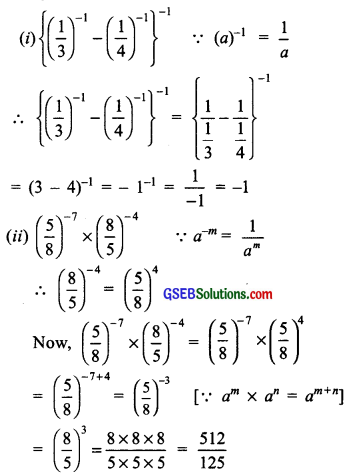Question 7.
Simplify:
(i) $$\frac{25 \times t^{-4}}{5^{-3} \times 10 \times t^{-8}}$$ (t ≠ 0)
(ii) $$\frac{3^{-5} \times 10^{-5} \times 125}{5^{-7} \times 6^{-5}}$$
Solution: